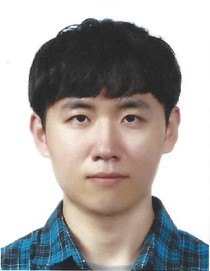# Deep Learning (딥러닝)

POSTECH
Enrollment is Closed

## 강좌 소개

최근 인공지능 분야에서 주목받고 있는 딥러닝을 이해하고 구현한다.

This course is designed to exploit and understand Artificial Intelligence, especially Deep Learning. Students are expected to learn theoretical backgrounds and their implementations of algorithms in Python. Starting from a basic machine learning, various kinds of neural networks will be intensively studied. Numerical Python coding is heavily required during lectures and homework assignments.

 [강좌 미리보기] week 1-2 [Optimization 1] 강좌 미리보기 week 1-3 [Optimization 2] 강좌 미리보기

## 강좌 목차

주차내용
1Introduction & OptimizationIntroduction
Optimization 1
Optimization 2
Optimization 3
Lab
2Machine LearningRegression
Classification: Perceptron 1
Classification: Perceptron 2
Classification: Logistic Regression 1
Classification: Logistic Regression 2
Lab
3
Machine Learning with TensorflowMachine Learning with Tensorflow 1
Machine Learning with Tensorflow 2
Regression & Classification with Tensorflow
Lab
4Machine Learning Optimization
Lab
Overfitting
Lab
 5 From Perceptron to MLP (ANN) Artificial Neural Networks 1 Artificial Neural Networks 2 Artificial Neural Networks 3 Lab Artificial Neural Networks Training 1 Artificial Neural Networks Training 2 Lab 6 ANN advanced ANN with Tensorflow 1 ANN with Tensorflow 2 Lab ANN advanced 1 ANN advanced 2 Lab 7 Autoencoder (AE) & Convolutional Neural Networks (CNN) Autoencoder 1 Autoencoder 2 Lab Convolution: 1D Convolution: 2D Convolution: Kernel 1 Convolution: Kernel 2 8 Convolutional Neural Networks (CNN) & Class Activation Map (CAM) Convolution: Padding and Stride Convolution: Pooling Convolutional Neural Network in Tensorflow Lab Class Activation Map (CAM) 1 Class Activation Map (CAM) 2 Lab
 9 Modern CNNs & Transfer Learning Modern CNNs Lab Transfer Learning Lab 10 Convolutional Autoencoders (CAE) & Fully Convolutional Networks (FCN) Convolutional Autoencoders (CAE) 1 Convolutional Autoencoders (CAE) 2 Lab Fully Convolutional Networks (FCN) Lab 11 Generative Adversarial Networks (GAN) Generative Adversarial Networks (GAN) 1 Generative Adversarial Networks (GAN) 2 Generative Adversarial Networks (GAN) 3 Generative Adversarial Networks (GAN) 4 12 Conditional GAN Conditional GAN Lab 13 Time Series Analysis Time Series Data 1 Time Series Data 2 Markov Chain Hidden Markov Model (HMM) and Kalman Filter Lab 14 Recurrent Neural Networks (RNN) Recurrent Neural Networks (RNN) 1 Recurrent Neural Networks (RNN) 2 Recurrent Neural Networks (RNN) 3 Recurrent Neural Networks (RNN) 4 Recurrent Neural Networks (RNN) 5 Recurrent Neural Networks (RNN) 6 Recurrent Neural Networks (RNN) 7 Lab

## Instructor### 이승철

포스텍 기계공학과 교수

UNIST 기계항공및원자력공학부 교수

UNIV. OF MICHIGAN DEPT. OF MECHANICAL ENGINEERING 박사 후 연구원

UNIV. OF MICHIGAN Mechanical Engineering 박사

## Staff### 나주원

포스텍 기계공학과

1. Course Number

DSC516
2. Classes Start

3. Classes End

4. Estimated Effort

3시간